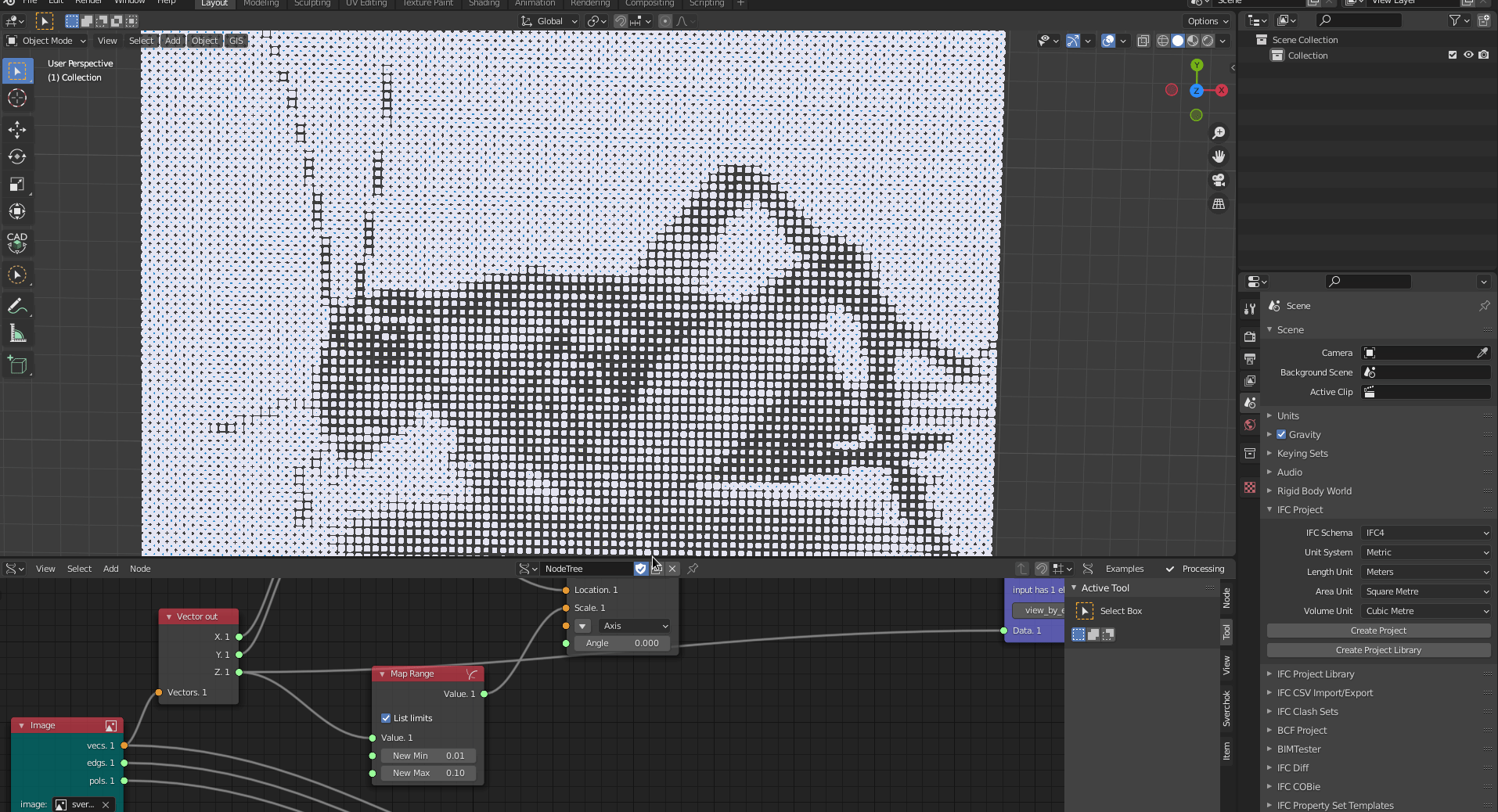## Image Sampler

Architects and designers have been explored the Image Sampler script for many years in parametric design. This script is simple and effective, and its purpose goes beyond architectural design. In architectural design, this script is often used for creating customised facade panels produced through digital fabrication using CNC machines.

In this example, we reproduce an image composed of circles that could be fabricated as a customised perforated facade panel.We can make this script in a few steps.

## Creating the Image

First, we load an image of a сверчок or Sverchok (cricket), which is our adorable mascot. We go to Blender and change the `Editor Type` to `Image Editor`, then choose the Sverchok image that you can download here.Then we load the image in Sverchok using the `Image` node (`Generator -> Image`).Now we need to define the image size in Sverchok. This image has 550x400 pixels. We need to use a size proportional to the original image. For instance, we can do that by dividing the original size by a given number. We use two `Scalar Math` nodes, one for the `Xvec` input with the number `550`, and another for the `Yvec` input with the number `400`, respectively, image width and height. Then, we use `A Number`(`Number -> A Number`) as a divisor with the number `6`.We can use a `Viewer Draw`(`Viz -> Viewer Draw`) to visualise the points. Also, we use `A Number` with the value `0.06` to define `Xstep` and `Ystep`.Then, we use `Vector Out` (`Vector -> Vector Out`) and `Vector In` (`Vector -> Vector In`) to deconstruct the vertices in the corresponding values, `X`, `Y`, `Z`. Because in the next step, we are going to remap the values for `Z`.We remap the `Z` value using `Map Range`(`Number -> Map Range`) checking the option `List Limits`, defining `New Min` as `0.01` and `New Max` as `0.02`. Then, we use `Matrix In` using `X` and `Y` from `Vector In` as the input `Location` and `Z` output as input `Scale`.We can finalise our image using a `Matrix Apply`(`Matrix -> Matrix Apply`) node with a `Circle` node as input. Then connect the output from `Matrix In` into the input `Matrix` from `Matrix Apply`. To visualise, use another `Viewer Draw`. Easy!Do not forget to make the group.## Creating the Panel

It is a good idea to create a panel defining its borders. This border makes it possible to fabricate in a Laser Cutter Machine.

First, we create a `Plane`(`Generator -> Plane`), using the image size from `Scalar Math` output for both `X` and `Y` as input of `SizeX` and `SizeY`. Then, we use A `Viewer Draw` node to visualise it.To resize the panel, we multiply its size by `0.06`. It is the same number used for the space between vertices.

To make it parametrically, we connect it to the same `A Number` node that defines the space between points into the `Scalar Math` node with the `Multiply` for `SizeX` and `SizeY` inputs.Now we need to centralise the plane within the circles. To do that, we use a `Scalar Math` node with the operator `Negate` from the `A Number` output that defines the space between points to make it a negative value. Then, we use a `Matrix In` node using the `Location` parameter with the `Negate` output, connecting it to the `Plane` into the `Matrix` input.To finalise the panel, we can regularise the panel borders, adding a value to both `X` and `Y` plane size from the node `Scalar Math (DIV)`. We can use the value `0.3` with an `A Number` node.Finally, we create a group with the panel nodes.## Exporting the SVG file

Now, we can save the panel in an SVG file, making it possible to send it to a laser cutter machine, for example. First, we create a `Path SVG`(`SVG -> Path SVG`) node with the circles from the `Matrix Apply` output. We click on `Fill/Stroke` to create a `Fill/Stroke` node. Then, we change the `Fill` parameter to `None` and `Stroke` parameter to `Flat`, defining the `Stroke Width` to `0.01`.Then, we use the `Mesh SVG`(`SVG -> Mesh SVG`) node to create the panel lines, connecting the `vertices` and `edges` from the `Plane` output. We click on `Fill/Stroke` to create a `Fill/Stroke` node. Then, we change the `Fill` parameter to `None` and `Stroke` parameter to `Flat`, defining the `Stroke Width` to `0.01`.We create two groups of SVG lines, one for `cutting` with the circles and the other with `sheet` with the panel. We use `SVG Group` (`SVG -> SVG Group`) to make it.We use a `List Join` (`List -> List Main -> List Join`) node to create a single list with both groups, then `SVG Document` to export it to SVG. We define an A4 landscape standard 297x210 with the `Scale` parameter as `50`. Then, we save the SVG by clicking on `Folder Path`, checking `Live Update`, clicking on `Open Server` to open the live preview.We can centralise the SVG by changing the `Offset` value from the `Group SVG` input. Then, we can click on the `Write` option in the `SVG Document` node to save it into a file.Do not forget to make the final group.Now we can open it in Inkscape. =)Have fun.